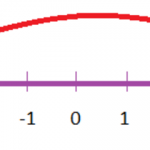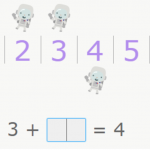Smartick is an advanced online program that teaches kids math and coding in only 15 min. a day

Content about adding and subtracting in elementary mathematics. Exercises, tutorials, and problems with adding and subtracting in elementary mathematics. Here you will find posts about adding and subtracting to make learning mathematics easier and more fun.

Mar30

## Learn How to Add Polynomials with Examples

What are polynomials? Before we start adding them, we need to know what they are and what properties they have. It’s important to understand what factors and degrees are, and how to order the terms. If you’re not sure about these concepts, you can read these previous posts about the properties of monomials and properties […]

Mar17In today’s blog post, we are going to look at integers: what they are and how to add and subtract them. Let’s start by understanding what integers really are: Integers are numbers that aren’t a fraction or a decimal. These numbers include natural numbers (1, 2, 3…), negative numbers (-1, -2, -3…) and zero (0). […]

Feb03

## Learn to Subtract with Remainders

Today, we will be learning to use “borrowing,” (sometimes called “regrouping”) in a subtraction problem. In order to do this we need to remember how the elements of a subtraction problem are placed: Place the number being subtracted under the number being subtracted from so that place values in the same column match. Subtract each […]

Jan16

## Adding with an Unknown QuantityIn this post, we’re going to explain what adding with an unknown quantity is and why it’s taught during the first years of Elementary school. Some people think it’s strange that children are taught such an important variable, like an unknown quantity, in addition problems shortly after the children have just started to get used […]

Dec03

## How to Solve Subtraction Problems

In this post, we are going to learn to analyze, think about, and solve problems that require subtraction. At Smartick, we believe that a student understands and knows math the best when they have the ability to solve problems correctly. Therefore, we are going to analyze 5 different problems that require subtraction to reach a […]

Nov28

## How to Solve Addition Problems

In this post, we are going to learn to analyze, think about, and solve addition problems. Usually, failure in mathematics comes from not understanding the problems that we are given. This is why we are going to analyze 5 problems which require addition to solve. Addition Problems nº 1 There were 8 cars in the […]